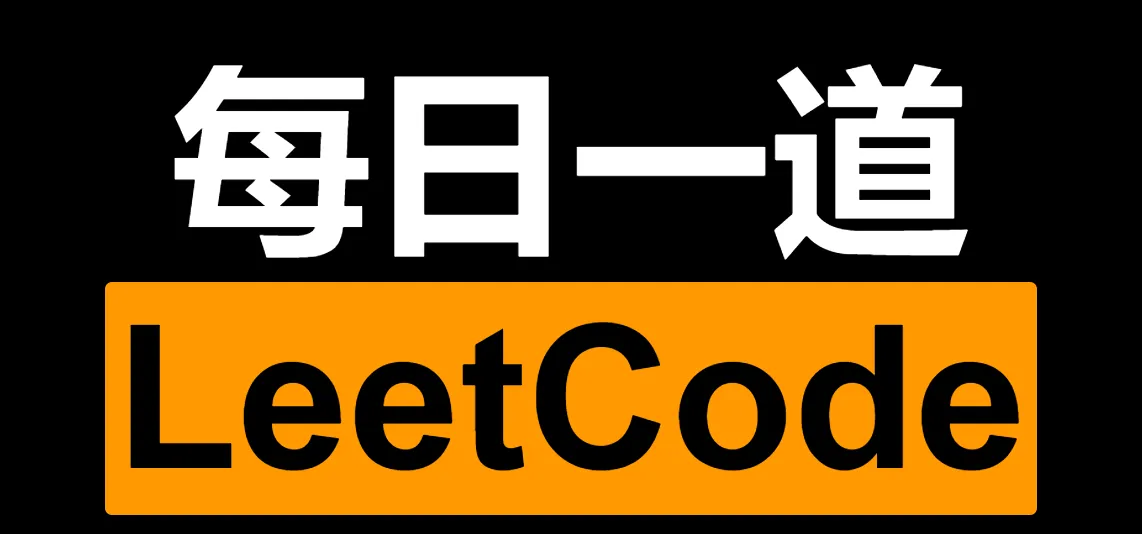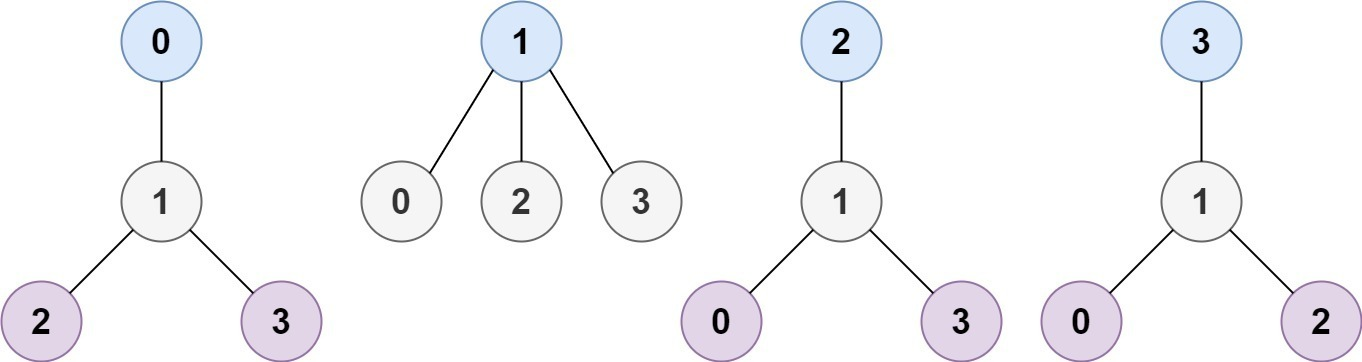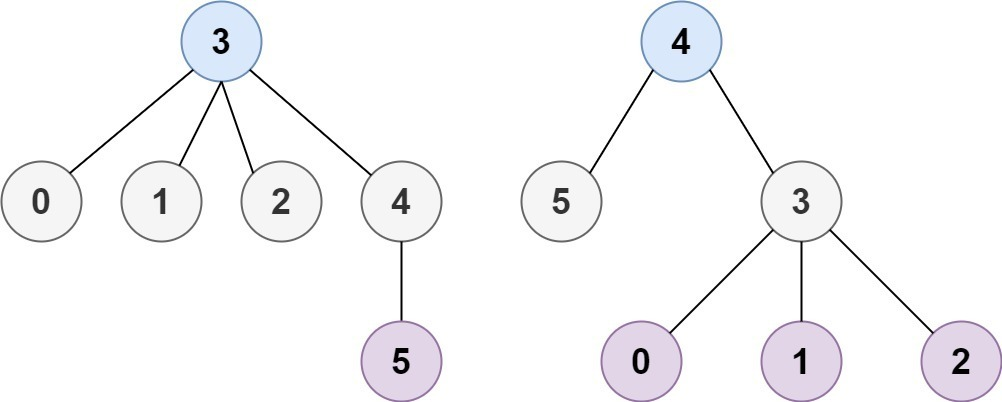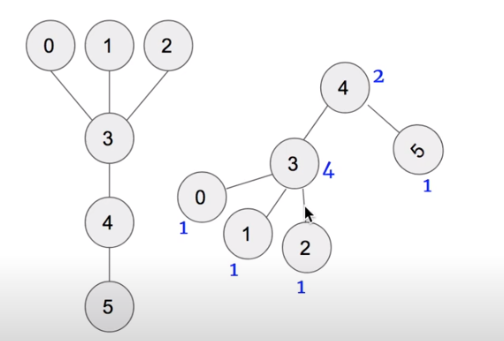# leetcode 310. Minimum Height Trees 最小高度树 (中等)

• 2022 年 6 月 13 日
• 本文字数：1405 字

阅读完需：约 5 分钟# 一、题目大意• 1 <= n <= 2 * 104

• edges.length == n - 1

• 0 <= ai, bi < n

• ai != bi

• 所有 (ai, bi) 互不相同

• 给定的输入 保证 是一棵树，并且 不会有重复的边

# 二、解题思路# 三、解题方法

## 3.1 Java 实现

public class Solution {    public List<Integer> findMinHeightTrees(int n, int[][] edges) {        List<Integer> ans = new ArrayList<>();        if (n == 1) {            ans.add(0);            return ans;        }        int[] degree = new int[n];        Map<Integer, List<Integer>> nodeMap = new HashMap<>();        for (int i = 0; i < n; i++) {            nodeMap.put(i, new ArrayList<>());        }        for (int[] edge : edges) {            nodeMap.get(edge).add(edge);            nodeMap.get(edge).add(edge);            degree[edge]++;            degree[edge]++;        }        Queue<Integer> queue = new LinkedList<>();        for (int i = 0; i < n; i++) {            if (degree[i] == 1) {                queue.add(i);            }        }        while (!queue.isEmpty()) {            List<Integer> list = new ArrayList<>();            int size = queue.size();            for (int i = 0; i < size; i++) {                int cur = queue.poll();                list.add(cur);                for (int tmp : nodeMap.get(cur)) {                    degree[tmp]--;                    if (degree[tmp] == 1) {                        queue.add(tmp);                    }                }            }            ans = list;        }        return ans;    }}

# 四、总结小记

• 2022/6/13 下班的时候爆雨停了## 评论